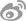•• 理论中国网   |
•"党的文献"公众号"黄城根下"公众号"党的历史"公众号
•（微博）党史网1993年被评为编审，并获国务院政府特殊津贴。曾被聘为中共中央文献研究会名誉理事、毛泽东思想生平研究分会名誉常务理事。在文献研究室工作期间，先后参加编辑《毛泽东农村调查文集》、《毛泽东书信选集》、《毛泽东早期文稿》，担任《建国以来毛泽东文稿》、《毛泽东选集》第二版、《毛泽东文集》、《毛泽东著作专题摘编》等多部著作的主编之一,并担任大型画册《毛泽东》的副主编，大型电视文献纪录片《毛泽东》的总监制之一。曾参与《毛泽东军事文集》、《缅怀毛泽东》、《毛泽东读书笔记》等著作，以及有关毛泽东的大量文稿的审稿工作。其间曾撰写多篇论文，参加中央宣传部文献研究室等单位举办的学术研讨会。在纪念毛泽东诞生120周之际，曾以《毛泽东思想当代价值论集》为名结集出版。

• 翻译
• C
• D
• F
• G
• H
• J
• L
• M
• O
• P
• Q
• S
• T
• W
• X
• Y
• Z
• C
• F
• G
• H
• J
• L
• P
• Q
• S
• T
• W
• X
• Y
• Z
• C
• D
• G
• H
• J
• L
• Q
• S
• W
• X
• Y
• Z